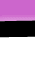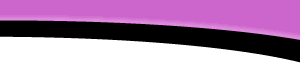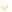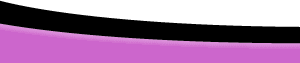HomeFAQsTutorialsResourcesHelpDreamweaverFAQ.com » Tutorials » JavaScript » Forms And Math Page 3# Forms and Math page 3

## Using the "to_currency()" Function with a Hidden Field

Consider the following simple form calculation, a hidden field is used to store the mathematical equivalent of "\$29.90", namely "29.9".

Enter # of widgets @ \$29.90 each

```<script language="JavaScript"><!--function calculate(){  with(document.maths){	  total.value = to_currency(widgets.value* hiddenField.value,"\$")  }}// --></script>

<form name="maths"><p> <input type="text" name="widgets">Enter # of widgets @\$29.90 each</p><p> <input type="text" name="total" value="Total"></p><input type="hidden" name="hiddenField" value="29.9"><input type="button" name="Button" value="Calculate" onclick="calculate()"></form> ```

At first glance, the function appears to be adequate for our use. But remember, whenever a form interacts with the user, great care must be taken to ensure usable input. The "to_currency()" function is invoked within the "calculate()" function, this will ensure that the user's entry is a Number. But we must consider other undesirable Number inputs, negative and floating point Numbers (non whole numbers such as 10.5). Of course it is up to the programmer to decide exactly what to do with these unsuitable entries. For the purposes of this tutorial, I will disallow negative entries, but I will allow the user to round a floating point entry upwards using the built-in window method "window.confirm()"

Try it, enter a non whole number above, then press Calculate.

Enter # of widgets @\$29.90 each

```<script language="JavaScript"><!--function calculate(){  with(document.maths){  if(widgets.value<0){	  alert("Positive numbers only, please"); 	  return;  }  if (widgets.value % 1!=0){	  if(window.confirm("Round up to "+Math.ceil(widgets.value)+"?"))
{		  widgets.value=Math.ceil(widgets.value);		  }	  else{		  return;	  }  }  total.value = to_currency(widgets.value* hiddenField.value,"\$");  }}// --></script> ```

if(widgets.value<0){
return;
}

If the value of widgets is less than zero, alert the user, terminate the function.

if (widgets.value % 1!=0){
if (window.confirm("Round up to "+Math.ceil(widgets.value)+"?")) {
widgets.value=Math.ceil(widgets.value);
}
else{
return;
}
}

If you divide the value of the widget by one and the remainder does not equal zero, then run the code that follows between the curly brackets:
{
If the user answers "yes" to the question: "Round up to [next higher whole number] ?", then
{ widgets value is rounded up to a whole number}
else if the user answers "no",
{terminate the function(return).}
}

Notice how the rounded up Number is included in the window confirmation message, Math.ceil(widgets.value). The message will vary according to the user's entry.Copyright © 2001-2021 DreamweaverFAQ.com All Rights Reserved.